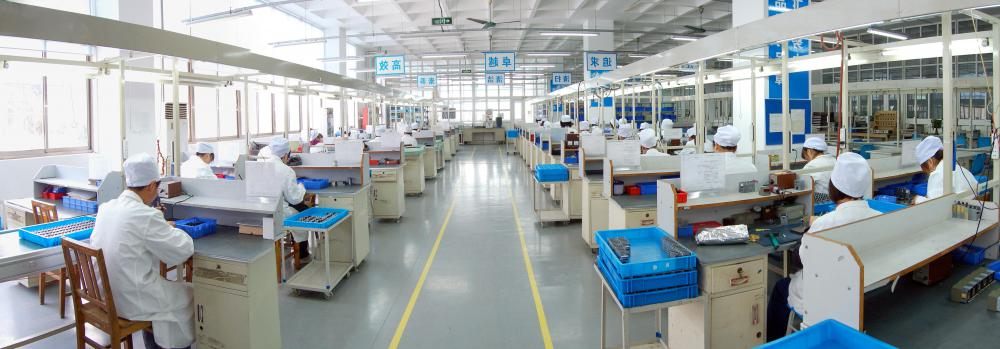# What Is Constant Marginal Cost?

Jim B.

Constant marginal cost is the total amount of cost it takes a business to produce a single unit of production, if that cost never changes. Since the cost is the same for every single unit produced, it is considered a constant. The variable part of the equation to estimate costs is the total volume of items that the company produces. As that amount changes, so too will the costs for the production order, even as the constant marginal cost remains unchanged.Constant marginal cost is the total amount of cost it takes a business to produce a single unit of production, if that cost never changes.

Companies that produce items in mass quantities must always be cognizant of the costs associated with production. Doing so requires coming up with methods for estimating these costs before production orders are filled. By performing these estimates, management can properly budget for any size order it may receive, all while making sure that the company's bottom line improves. It is important to understand the concept of constant marginal cost in order for companies to set up production systems that allow them to produce goods at a steady cost rate no matter the size of the order.When factory employees are paid per unit produced, their wages can be included in constant marginal cost.

Trying to understand this concept can be tricky, since the name implies two seemingly opposite things working against each other. The marginal cost is the cost it takes to produce a single item. If that cost is constant, it means that one item will cost exactly the same whether it is the first item being produced for an order or the millionth. For example, if it takes \$100 US Dollars (USD) for a company to make a single item, and that remains unchanged for an entire order, the constant marginal cost is \$100 USD.

It is also important to separate this cost from fixed costs. Fixed costs are those costs attached to production no matter what the scenario might be. For example, simply turning the lights on in a factory costs the parent company a certain amount of money. Those costs will be incurred every time production is underway. The constant marginal cost, even as it remains the same, will be multiplied to the amount of items produced to yield the variable costs, which, unlike fixed costs, change depending on the size of the order.

When estimating costs for production, the constant marginal cost is often part of a linear cost function. Total costs will be equal to fixed costs added to variable costs, which, as mentioned above, is dependent on the marginal cost. Such a function is linear because the marginal cost is constant, causing the values for the number of items produced and total costs, when shown on a graph, to form a straight line. This does not occur when the marginal cost varies depending upon the amount of items being produced.

## You might also LikeMarkerrag

This concept seems highly subjective to external forces. The cost is supposed to be constant, but what happens if, say, the cost of raw materials increases? The term "constant" might not be applicable, after all.